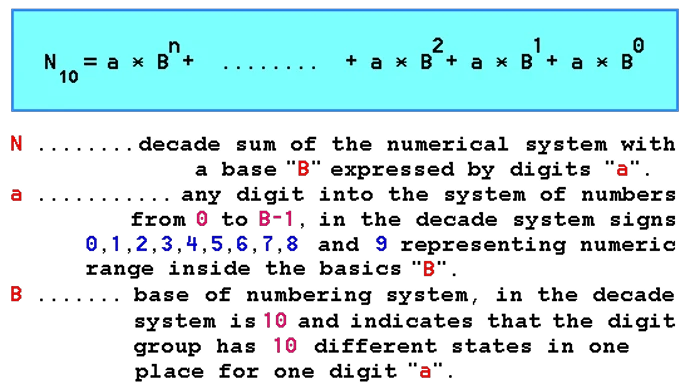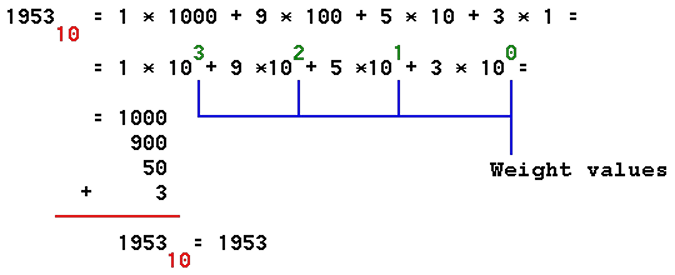# 2. BASIC OF DIGITAL COMPUTER SYSTEMDigital Computer System (DCS) is used to assist man in solving tasks and problems in any area of its operations. Speed and a large number of operations per unit time to use a Digital Computer System (DCS) to solve very complex tasks that a man without a computer very long service solved.

Of particular importance is the property of DCS to quickly perform mathematical operations therein adapted system of numbers using electronic components and devices created specifically for this purpose. It must therefore be familiar with the basics of these components and numbering systems that work in the computer and work with it are used.

# 2.1. NUMBER SYSTEMSNumber system is a way of marking or expressing numbers, strings or names. In parallel with the development of human history through the letters have evolved and different number systems that are shared by the structure:

```        1.) additive,

Additive system is a series of characters in which the number of characters equal to the sum of which it is composed, for example, as the ancient Romans:XXXVII = 10 + 10 + 10 + 5 + 2 = 37

These systems did not allow for such an operation is possible additive-multiplicative number systems, in which individual numbers (digits) depict the size of the individual groups of a given string with which to multiply and all groups are added:"one hundred and forty five" = 1 • 100 + 4 • 10 + 5 • 1 = 145

The basis of additive-multiplicative number system is the BASE, which enters as a component in multiplicand marks, names or numbers. Today it is in general use DECADE NUMBER SYSTEM, additive-multiplicative number system with BASE of ten (10).

In general, the number of 'N' in the additive-multiplicative system with a base 'B' can be written as:Given expression to calculate the value of the decade 'N' of any number system.People counted by decade system of numbers and often do not think that it was created on the basis of ten human fingers with which he assisted in calculations. In some tribes in remote forests, in some way isolated from the present civilization is encountered and the count system based on 20th Query: On what basis they used a system based on 20? Perhaps the most fortunate ones who lived by the principle counted, one, two, three, and a lot. Often in the literature uses the term 'decimal number system'. The terms 'deka' and 'deci' have the same linguistic background, but as each number with a decimal point is called the decimal author of these pages is acceptable name used. Besides, according to the SI system of units 'deka' means 10 (ten) as 'deci' means 1/10 (one tenth), and BASE is 10 (ten). Binary, octal and hex numbers can also be a decimal, that is a decimal point number.

Use positional labeling issues such as the number 1953 has four digits each, depending on the place where the means of units, tens, hundreds, etc. Each digit joins her WEIGHT, which depends on its place in the number. Lowest weight is on the right end digit number and the greatest weight has digit on the left end of the number.

Decade number is interpreted as follows:The basis of the number 10 and the weighted value of the digit is the exponent basis in accordance with the numbers from a distance of minimum weight, starting with the value ' 0 ' onwards.

On the negative exponent can display numbers less than one, such as:0.1210 = 1 • 10-1 + 2 • 10-2

Often in everyday practice describing events which is the basis of counting different, for example, or the sun is gone, dead or alive, wet or dry, and the like. The descriptions associated with two different states. Electronics is no exception. It is very complicated electronic circuitry that would signal amplitude shown in Figure 1.1.2 ten levels differed in size. The system would be resistant to any interference that could alter amplitude.

It is easier to define TWO situations, impulse has (positive pulse) or absent (absence of pulse or negative pulse), regardless of its shape and size. Symbolic marks of existence impulse is "1" and the absence of designation is "0". Electronic circuit that separates the existence and non-existence of pulses is much simpler than the complex that must distinguish the different signal size, and therefore the computer to design a computational and logical operations performed with a numbering system that uses the digits "0" and "1" and has a base ' 2 '.

Such a system is called the BINARY NUMBER SYSTEM in which the digits can occur "0" or "1", which represents a 50% probable event of the occurrence of one of the digits. That means that the amount of information contains the digits is 1 bit.

In addition to the description of the numbers that have no basis label implies 10 basis, and the numbers of other numeric system will mark their accompanying basis or at the end of the record the initial system to which it belongs (10B, 16H ...).Citing of this page: Radic, Drago. " IT - Informatics Alphabet " Split-Croatia. {Date of access}. . Copyright © by Drago Radic. All rights reserved. | Disclaimer